• 今天，以求解数组最大值最小值为例，带领大家学习一下数组的基本应用。首先是定义一个数组，然后再用Rnd随机函数给数组元素赋值，接着使用循环& 选择结构来实现求解。VBde1Private Sub Command1_Click()'求解...


在VB编程中，数组占据着相当重要的作用，往往结合循环控制结构、函数过程出现在程序代码中。今天，以求解数组最大值、最小值为例，带领大家学习一下数组的基本应用。首先是定义一个数组，然后再用Rnd随机函数给数组元素赋值，接着使用循环 & 选择结构来实现求解。VBde1Private Sub Command1_Click() '求解最大值与最小值Dim a%(1 To 20), max%, min%, i& '定义数组与变量Randomize '产生随机数种子max = a(1)min = a(1)For i = 1 To 20    a(i) = Int(Rnd * 121 + 30) '产生30--150之间的随机整数    Print a(i);    If i Mod 5 = 0 Then Print '每行输出5个数    If max < a(i) Then max = a(i)    If min > a(i) Then min = a(i)Next iPrint "------------------------------------------------"Print "最大值：Max值为"; max & ",最小值Min值为" & minEnd Sub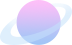问题来了！上面的代码运行后，我们会发现问题：最大值求出来的没有问题，但是最小值得到的是0，这是哪儿出问题了呢？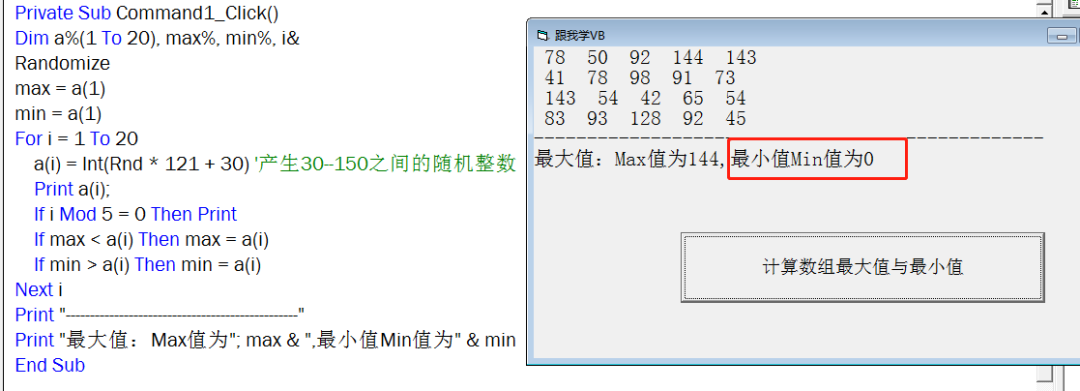问题解决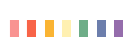遇到问题就要及时解决，这样才会不断进步，不断提高！如果一个人在学习的过程中，没有遇到过任何问题，那显然是不可能的，尤其是在编程语言的学习中，逻辑一定要清晰，知识内容要熟练掌握。问题产生原因：min = a(1)If min > a(i) Then min = a(i)这两句代码专门抽起来，帮助大家更好的理解。a(1)在数组没有赋值前，其值是0，所有min=0;在数组赋值中，我们知道是产生的30--150之间的随机整数，所有任何一个数组元素其值一定是>=30的。很明显，min是0，在执行If语句时，If min > a(i) 怎么都不会成立，所以Then后面自然也就不执行，最后得到的就是0了。问题解决：在定义Min时，我们要定义为一个大的数，这样在程序中与数组中的元素一一比较，小的元素值赋值给Min，这样才是正确的。改进后的代码Private Sub Command1_Click()Dim a%(1 To 20), max%, min%, i&Randomizemax = a(1)min = 150For i = 1 To 20    a(i) = Int(Rnd * 121 + 30) '产生30--150之间的随机整数    Print a(i);    If i Mod 5 = 0 Then Print    If max < a(i) Then max = a(i)    If min > a(i) Then min = a(i)Next iPrint "------------------------------------------------"Print "最大值：Max值为"; max & ",最小值Min值为" & minEnd Sub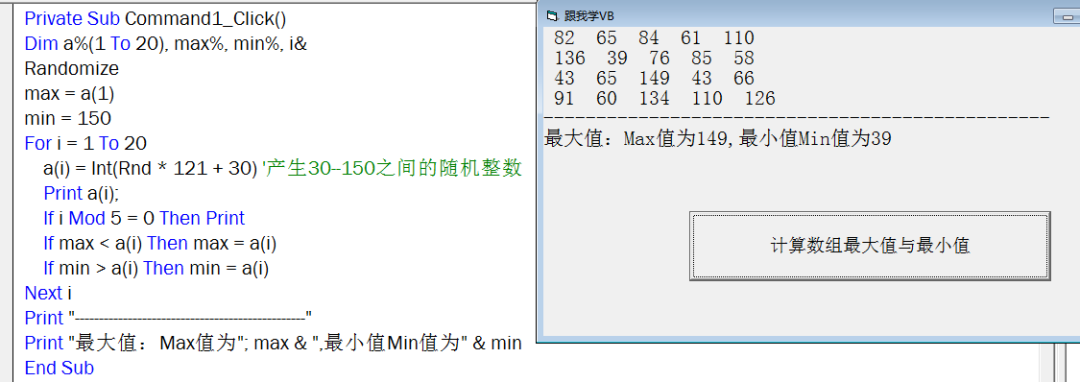修改后再运行，结果正确无误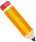答疑解惑学习VB中有问题可以长按识别下图添加老师微信号，学习问题在线答疑。展开全文• vararr=[2,1,6,99,101,4,1,23]; varnewArr=[]; for(vari=0;...选择排序的思想var max = arr;var min = arr;for(var i = 1; i < arr.length; i++) {if(max < arr[i]){max = arr[i];}i...
var arr = [2, 1, 6, 99, 101, 4, 1, 23];     var newArr = [];     for(var i = 0; i 2.选择排序的思想var max = arr;var min = arr;for(var i = 1; i < arr.length; i++) {if(max < arr[i]){max = arr[i];}if(min > arr[i]){min = arr[i];}}console.log("数组的最大值为："+ max +"，最小值为："+ min);3.使用ES5中提供的数组内置map方法，原理还是遍历整个数组，在新数组中再进行操作var newArr = [];var res = arr.map(function(val, idx, self) {newArr.push(val);})newArr.sort(function(a, b) {return a-b;})console.log("数组的最大值为："+ newArr[newArr.length - 1] +"，最小值为："+ newArr);4.使用ES5中提供的数组内置reduce方法，这种方法较难理解，我们结合代码理解var max = arr.reduce(function(prev, val, idx, self) {if(prev > val){return prev;}else{return val;}}, arr)var min = arr.reduce(function(prev, val, idx, self) {if(prev < val){return prev;}else{return val;}}, arr)console.log("数组的最大值为："+ max +"，最小值为："+ min);方法还有很多，后续可更，也欢迎开发朋友们来讨论其他方法！
展开全文•  * 在n行n列的数组里面按一下要求选出两个数。首先从每行选出最大的数，再从n个最大的数里面选出一个最小的数；  * 其次，从每行选出最小的数，再从选出的最小的数里面选出一个最大的数。  * */ #include #...
/*
* 在n行n列的数组里面按一下要求选出两个数。首先从每行选出最大的数，再从n个最大的数里面选出一个最小的数；
* 其次，从每行选出最小的数，再从选出的最小的数里面选出一个最大的数。
* */
#include <stdio.h>
#include <conio.h>
#include<stdlib.h >
#define MAXN 20
int a[MAXN][MAXN] ;
void main(void)
{int max ; /*save max value*/int min ; /*save min value*/int row , col , n ;system("cls");printf(" please input the elements of the matrix : \n ") ;
/* 输入方阵的阶次*/scanf(" %d " , &n ) ;printf(" please input the order of the matrix \n from a to a[%d][%d] : \n " , n-1 , n-1 ) ;for(row = 0 ; row < n ; row ++){for(col = 0 ; col < n ; col ++){scanf( " %d" ,  &a[row][col] ) ;}}for(max = a , row = 0 ; row < n ; row ++){/* choose the min value from row*/for(min = a[row] , col = 0 ; col < n ; col ++){if(min > a[row][col])
/*save min value from row */{min = a[row][col] ;}if( min > max){max = min ;}}}printf(" the maxxnum of minxnum is %d :\n  " , max ) ;for(min = a , row = 0 ; row < n; row ++){for( max = a[row] , col = 0 ; col < n ; col ++){if( min > a [row][col])
/* save the max value every row*/{max = min ;}if(max < min ){max = min ;}}}printf(" the minxnum of maxxnum is : %d \t " , min ) ;getch() ;
}
展开全文matrix input system
• 此文章为博主在网上摘抄，如有侵权请告知，谢谢C++ STL之min_element()与max_element（）（取...作用：返回容器中最小值最大值。max_element(first,end,cmp);其中cmp为可选择参数； 1 #include&lt;iostrea...
此文章为博主在网上摘抄，如有侵权请告知，谢谢C++ STL之min_element()与max_element（）（取容器中的最大最小值）min_element()和max_element头文件：#include<algorithm>作用：返回容器中最小值和最大值。max_element(first,end,cmp);其中cmp为可选择参数； 1 #include<iostream>   2 #include<algorithm>   3 using namespace std;   4 bool cmp(int a,int b)   5 {   6       return a<b;   7 }   8 int main()   9 {  10       int num[]={2,3,1,6,4,5};  11       cout<<"最小值是 "<<*min_element(num,num+6)<<endl;  12       cout<<"最大值是 "<<*max_element(num,num+6)<<endl;  13       cout<<"最小值是 "<<*min_element(num,num+6,cmp)<<endl;  14       cout<<"最大值是 "<<*max_element(num,num+6,cmp)<<endl;  15       return 0;   16 }
展开全文• 打印数组最大值 class Demo { public static int getMax(int[] arr) { int max =0; for(int x= 0;x;x++)冒泡排序 class
• 我们看一下如何为数组取最大值最小值。最笨的方法估计是这样： 01.Array.prototype.max = function() { 02.var max = this; 03.var len = this.length; 04.for (var i = 1; ...
• System.out.println("最大数为:"); System.out.println(num); System.out.println("最小数为:"); System.out.println(num); System.out.println("差值为:"); j=num-num; System.out....java
• 原型是个好东西，通常除了Object的...我们看一下如何为数组取最大值最小值。最笨的方法估计是这样： Array.prototype.max = function () { var max = this; var len = this.length; for (var i = 1;...
• namespace 一维数组最大值最小值 { class Program { static void Main(string[] args) { int[] quene = new int {89,78,65,52,90,92,73,85,91,95 }; int max, min; max = min = quene; for (int i ...
• 给定一个无序可重复整数数组，用最简洁的代码求出该数组最小值最大值。 给定一个无序整数数组，用最简洁的代码求出该数组最小值最大值。 解题思路：两种解法，循环查询和递归查询，因为是无序可重复，递归在...java 算法
• 数组 元素类型[] 数组名 = new 元素类型[元素个数或数组长度]; int [] arr = new int ; //需要一个容器，但是不明确容器的具体数据。 int [] arr = new int [] {3,1,4,5,} //需要一个容器，存储已知的具体...java
• //数组中排序int in[] = {1,6,5,8,9};Arrays.sort(in);...}//数组中取最大值最小值int arr[] = {6,3,8,5,7,4,1};int min2 = arr;int temp = 0;for(int i=1;iif(arr[i]temp =min2;min2 = ar...
• class ArrayToolDemo{/***/public static void main(String[] args){int[] arr = {3,2,5,1,6,8,7,4};//定义一个数组int max = ArrayTool....//将获取的数组最大值赋给max。int min = ArrayTool.getMin(arr);//将...
• 参考《数据结构与算法分析》186页，...（同样，第k个最大值为第size - k + 1个最小值） #include #include #define cutOff 3 using namespace std; //三数中值分割方法 int Media3(vectorint>& vecUnsort, int
• Medium级别的题：给定整数数组nums，每次操作允许你选择一个值，把它变为任何一个值，最多进行3次这样的操作，使得变换后数组最大值最小值的差值尽可能小，问这个最小值是多少？数据范围，数组长度1<=nums....
• 获取数组的最值(最大值最小值)思路： 1、获取最值需要进行比较，每一次比较都会有一个较大的值，因为该 值不确定，需要一个变量进行临储。2、让数组中的每一个元素都和这个变量中的值进行比较，如果大于了 变量中...
• memset赋值赋的是ASSCII码转为二进制赋值 比如 memset(,0xff,sizeof()),0xff转为二进制11111111，...而OIER通常都希望能够通过memset赋给数组一个最大值 ## 如何定义这个无穷大 这个主要还是看数据范围。 如果直接...
• public class max { public static void main(String[] args) { int[] nums = {1,19,20,3,6,9,30};... // 输出最大值 int max = nums; for (int i = 0;i < nums.length;i++){ if(nums[i] > max){ .java 排序算法 算法
• 获取数组的最值（最大值最小值） 思路： 1、获取最值需要进行比较，每一次比较都会有一个较大的值，因为该 值不确定，需要一个变量进行临储。 2、让数组中的每一个元素都和这个变量中的值进行比较，如果大于了 ...
• 获取数组的最值(最大值最小值)思路： 1、获取最值需要进行比较，每一次比较都会有一个较大的值，因为该 值不确定，需要一个变量进行临储。2、让数组中的每一个元素都和这个变量中的值进行比较，如果大于了 变量中...
• 获取数组的最值(最大值最小值)思路： 1、获取最值需要进行比较，每一次比较都会有一个较大的值，因为该 值不确定，需要一个变量进行临储。2、让数组中的每一个元素都和这个变量中的值进行比较，如果大于了 变量中...
• class ArrayTool { public int getMax(int[] arr) { int max=0; for(int i=0;i;i++) { if(arr[i]>arr[max]) {class
• 请你返回三次操作后， nums中最大值最小值的差的最小值。 示例 1： 输入：nums = [5,3,2,4] 输出：0 解释：将数组 [5,3,2,4] 变成 [2,2,2,2]. 最大值最小值的差为 2-2 = 0 。 示例 2： 输入：nums = [1,5,0...
• 三次操作后最大值最小值的最小差给你一个数组 nums ，每次操作你可以选择 nums 中的任意一个数字并将它改成任意值。请你返回三次操作后， nums 中最大值最小值的差的最小值。示例 1：输入：nums = [5,3,2,4] ...
• 数组的一些运算获取数组最大值最小值及总和数组的顺序查找二分法查找冒泡排序直接选择排序数组的反转 获取数组最大值最小值及总和 public class GetArrayMaxMinIndex { public static void main(String[] args)...
• * 对int数组进行排序，使第1个是最大，第2个是最小的 * 第三个是次大的，第四个是次小的，以此类推 * 例如a={3,7,1,9,10,2,6,4,8,5} * 则输出结果为{10,1,9,2,8,3,7,4,6,5} */ #include&lt;stdio...算法
• 问题描述：寻找数组中的最大值最小值。 分析：这个问题实际中用的非常多，需要我们好好研究下。我在之前的博客中曾提到过一个线性时间选择算法用来求解TopK问题，同样的这个也可以使用那个算法，实际就是TOP1和...
• 一般一开始想到的办法就是一次循环，记录下最大值最小值。或者就是用两次冒泡，找到最大值和次大值。这两种方法实践复杂度差不多都是O(2n)，如果数组很长，效率还是不够高的。假如先排序，再选择最大的两个值，这...数据结构...